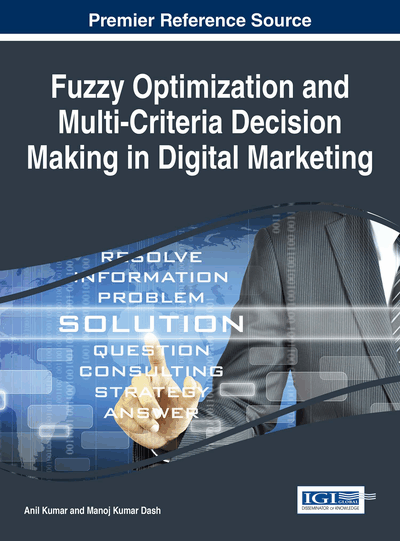# Fuzzy and Soft Poly-Optimization in the Digital Environment: Examples

Wojciech Tarnowski (Koszalin University of Technology, Poland)
DOI: 10.4018/978-1-4666-8808-7.ch009
Available
\$37.50
No Current Special Offers

## Abstract

TThe standard optimality problem is when the triad is given: <x, F(x), O(x)>, where x is the decision variables vector, F(x) is an overall optimality criterion and O(x) is a set of constraints, defined in a fully mathematical form. But in the real practice in business, logistics, medicine, and even in engineering – where a decision–maker has for his/her disposal comparatively good unique mathematical models – it is very rarely. Another trouble may be, the computations are time consuming and/or algorithms do not converged satisfactory. In this chapter examples of two non-standard techniques of poly-optimization (Multi Attribute Decision Making, Multi Criteria Decision Making) are presented. The first is a dialog procedure of finding satisfactory solution on hierarchical play of constraints, with extensive computer simulations, what is called here as a soft optimization. The other is a standard poly-optimization on fuzzy mathematical definition of criteria and of a model. A few examples are given.
Chapter Preview
Top

## Introduction

The topic of this chapter is the computer aided decision making. It is related to economy, medicine, managing etc., but typical fields are logistics and engineering, where mathematical models have been extensively developed. Typically, useful models there are in:

• Engineering design,

• Scheduling, timetable designing,

• Planning of operations,

• Resources deployment,

• Management, logistics.

Not as it has been in the past, nowadays a human decision-maker is facing a pressure to choose a variant from a very numerous (if not from a continuous) set of possible variants. The decision itself is still an arbitrary human act – however it may and should be aided by formal analytical procedures.

A digital environment and computer systems are broadly capable to formalize heuristic abilities of human decision makers. Artificial Intelligence methods and techniques may extensively be applied to make decisions better – more rational and compatible to human intuition and expertise.

Generally, Computer Aiding Systems:

• Immensely accelerate calculations, visualization and searching data,

• Effectively process complex data (like in ANN – Artificial Neural Networks),

• Provide intelligent post-processing of results as graphical presentations, adequate to human cognition abilities, and easy for assimilation and evaluation,

In the CAD approach to design, at the historical beginning, optimization was the paradigm , . It happened also in the general decision making philosophy. But there has been an objection to the necessity of arbitrary formulation of one unique optimality function. Then the multi–attribute approach was proposed with a scalar compromise optimality function, and also the poly–optimal description of decision problems. Again, the drawback was in the time–consuming computations and typically great dimensions of the Pareto solutions set (see Example 3). Some techniques to reduce such set were proposed (Osyczka, Montusiewicz ). If there were more than three criteria, the set was difficult for analysis (see Example 3 on a gear transmission). Besides, a human operator had to take the final decision arbitrarily, and this caused other objections.

As for now, optimization techniques are not very popular in real engineering, although they are taught at universities and are recognized as very useful in design and in management. Probably the main reasons are that mathematical models of objects are difficult to determine and the criteria may not be precisely defined.

The goal of this paper is to present two non-standard approaches to resolve difficulties in defining the optimization problems:

• 1.

With the fuzzy description,

• 2.

When criteria of optimization are not explicit defined,

and to demonstrate, that they may be easily applied by non-experts in MATLAB environment.

### Notions

The following terms will be used:

• Decision Variables: parameters that are to be calculated in (poly-)optimization procedure;

• Variant: a specific vector of decision variables; or a specific vector in performance parameters space;

• Constraints: limits imposed on admissible variant decision variables and for performance parameters; they define admissible variants;

• Criteria: performance parameters, which must be at minimum or maximum;

• Optimization, Optimality Problem: searching for optimal decision variables;

• Solution of the Optimality Problem: the optimal variant;

• Compromise Variant (Equivalent Names: A Trade-Off Variant, Pareto-Optimal Variant; A Poly-Optimal Variant): such permitted variant, which may not be improved on one criterion without violation on another criterion;

• Solution of the Poly-Optimization (MADM, MCDM): set of compromise variants.

## Complete Chapter List

Search this Book:
Reset